## On Generalized Dirac's Equation

Delso J

Bachelor's Degree in Physics by Zaragoza University, Spain

Article
Article Info
Author Info
Figures & Data

## Abstract

In four dimensions, the Minkowski metric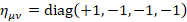leads to the 16 dimensional Clifford algebra C(1,3), Dirac equation is using four of these 16 matrices that form a basis of this algebra, a new operator is defined using all of these matrices and also generalized for a curved space

## Multilevel operator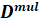We are using Pauli matrices σ, electromagnetic four-potential Aμ and charge e with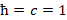.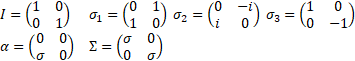(1)

In four dimensions, Minkowski's metric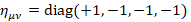leads to the Clifford algebra C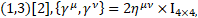Dirac matrices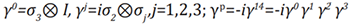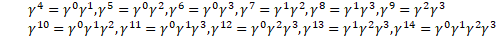(2)

Multilevel operator Dn acts on level n, n is the number of matrices in the product of the algebra members, for example, D3 acts on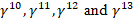. Total multilevel operator Dmul = D0 +D1 +D2 +D3 +D4, the action of Dmul on the spinor function vanishes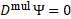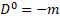(4)(5)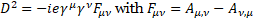(6)(7)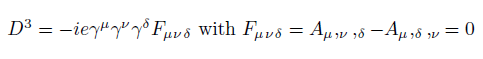(8)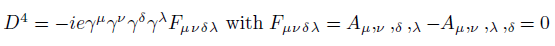(9)

Multilevel operator Dmul(ημν ,Aμ ,e) can be generalized for a curved space with four-potential P, field charge q and covariant derivative (;μ) instead of derivative (,μ) in the definition of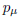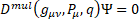(10)(11)(12)(13)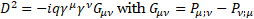(14)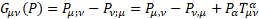(15)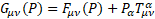(16)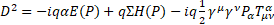(17)

For gravity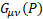is the new gravitomagnetic tensor.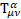is the torsion tensor .with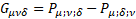(18)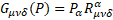with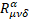the Riemann-Christoffel tensor .                                                                                                (19)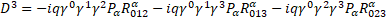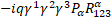(20)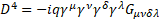with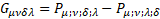(21)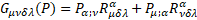(22)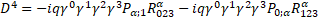(23)

## Conclusions

Multilevel operator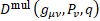has been generalized for a curved space with a general four-potential P. For gravityis the new gravitomagnetic tensor and torsion tensorappears in its definition.

In a flat space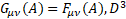and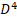operators vanish. In a curved space the curvature tensor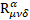appears in levels 3 and 4.

The appearance of torsion tensorand curvature tensorin multilevel operatormeans that this operator is a fundamental operator in Quantum Field Theory.

## References

1. Marian Fecko (2006) Differential Geometry and Lie Groups for Physicists. Cambridge University Press 637.
2. Marian Fecko (2006) Differential Geometry and Lie Groups for Physicists. Cambridge University Press 650-652.
3. Marian Fecko (2006) Differential Geometry and Lie Groups for Physicists. Cambridge University Press 380.
4. Marian Fecko (2006) Differential Geometry and Lie Groups for Physicists. Cambridge University Press 384.
5. Marian Fecko (2006 Differential Geometry and Lie Groups for Physicists. Cambridge University Press 404.

#### Article Type

Short Communication

#### Publication history

Accepted date: January 21, 2023
Published date: January 31, 2023

©2023 Delso J. This is an open-access article distributed under the terms of the Creative Commons Attribution License, which permits unrestricted use, distribution, and reproduction in any medium, provided the original author and source are credited.

#### Citation

Delso J (2023) On Generalized Dirac's Equation. OSP Journal of Physics and Astronom 4: JPA-4-142.

#### Jesus Delso Lapuerta

Bachelor's Degree in Physics by Zaragoza University, Spain. jesus.delso@gmail.com

No Figures